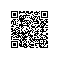# 《数值分析（原书第2版）》—— 2.6　用于对称正定矩阵的方法

### 2.6　用于对称正定矩阵的方法

#### 2.6.1　对称正定矩阵

25是对称正定矩阵.

25x1
x2
=2x21+4x1x2+5x22
=2(x1+x2)2+3x22该式子总是非负，而且不可能为0，除非x2=0以及x1+x2=0，而这两个条件放在一起意味着x=0.

45不是正定矩阵.

117xTAx=［x1　x2］24
45x1
x2
=2x21+8x1x2+5x22
=2(x21+4x1x2)+5x22
=2(x1+2x2)2-8x22+5x22
=2(x1+2x2)2-3x22例如，令x1=-2，x2=1，使得结果小于0，和正定矩阵的定义矛盾.

a21a22a23a24
a31a32a33a34
a41a42a43a44是对称正定矩阵，则a22a23
a32a33也是对称正定矩阵.118
2.6.2　楚列斯基分解

bc从对称正定矩阵的性质3我们知道a>0.并且我们知道矩阵A的值ac-b2是正的，这是由于矩阵的值为对应特征值的乘积，从性质1知道所有特征值都是正数，所以乘积也是正数.使用上三角矩阵将矩阵A写做A=RTR意味着ab
bc=a0
uvau
0v=aua
uau2+v2我们希望检查如上的分解是否可能.从我们对于矩阵的值的了解，左右两侧进行比较得到u=b/a以及v2=c-u2.注意，我们知道v2=c-(b/a)2=c-b2/a＞0.这也验证了v是一个实数，楚列斯基分解如下A=ab
bc=a0
bac-b2/aaba
0c-b2/a=RTR对于2×2对称正定矩阵成立.楚列斯基分解不唯一，很显然，我们同样可以选择c-b2/a负的平方根作为v.

bC

0
I
0

119得到ST1|0　…　0
0
A1
0S=a|0　…　0

uI1|0　…　0
0
A1
0a|uT
0
I
0
=a|bT

buuT+A1
=A　　注意到A1对称正定.这是由于，根据性质2，如下矩阵也是对称正定的1|0　…　0
0
A1
0=(ST)-1AS-1因而根据性质3，（n-1）×（n-1）主子矩阵A1也是对称正定的.通过归纳假设，A1=VTV，其中V是上三角矩阵.最后，定义上三角矩阵R=a|uT
0
V
0并检查RTR=a|0　…　0
uVT
a|uT
0
V
0=a|bT

buuT+VTV
=A完成证明.

结果中矩阵R是上三角矩阵，满足A=RTR.

-22-4
2-411R的最上面一行是R11=a11=2，其他元素是R1,2∶3=［-2,2］/R11=［-1,1］:R=2|-1　1

1-11得到|[-]
2-4
-411-|[-]
1-1
-11=|[-]
1-3
-310现在我们在2×2的子矩阵中重复这个过程，找到R22=1以及R23=-3/1=-3:R=　2[|]-1[-]　1
1[|]-3[-]

01-3
001　　对于对称正定矩阵A，求解Ax=b，和LU分解的方式相同.既然A=RTR是两个三角矩阵的乘积，我们需要求解下三角矩阵系统RTc=b和上三角系统Rx=c来求解x.
2.6.3　共轭梯度方法

=Axk+rk因而对k进行归纳得到rk=b-Axk.最后，变量dk表示用于更新xk得到改进的xk+1时所使用的新的搜索方向.

b-Axk+1=b-Axk-αkAdk
rk+1=rk-αkAdk
0=dTkrk+1=dTkrk-αkdTkAdk
αk=dTkrkdTkAdk这和算法中所写的αk不同，但是注意到dk-1和rk正交，我们有dk-rk=βk-1dk-1
rTkdk-rTkrk=0使得重写rTkdk=rTkrk成立.第二，选择系数βk，以保证dk两两A共轭:dk+1=rk+1+βkdk
0=dTkAdk+1=dTkArk+1+βkdTkAdk
βk=-dTkArk+1dTkAdk如下面的（2.47）所示，对于βk，可以重写为算法中的简单形式.

25u
v=6
3
123使用上面的算法x0=0
0,r0=d0=6
3
α0=6
3T6
36
3T22
256
3=456·18+3·27=521
x1=0
0+5216
3=10/7
5/7
r1=6
3-52118
27=121/7
-2/7
β0=rT1r1rT0r0=144·5/4936+9=1649
d1=121/7
-2/7+16496
3=180/49
-120/49
α1=12/7
-24/7T12/7
-24/7180/49
-120/49T22
25180/49
-120/49=710
x2=10/7
5/7+710180/49
-120/49=4
-1
r2=121/7
-2/7-71022
25180/49
-120/49=0
0由于r2=b-Ax2=0，解是x2=［4,-1］.

2.6.4　预条件

n×n的线性方程组Ax=b的预条件形式是M-1Ax=M-1b其中M是可逆的n×n矩阵，称为预条件子.我们所要做的就是在方程两侧左乘上该矩阵.预条件矩阵试图对矩阵A逆转，从而可以有效地降低问题的条件数.概念上来讲，它试图同时做两件事:矩阵M应该（1）和矩阵A足够接近，（2）容易求逆.这两个目的常常彼此对立.

xk+1=xk+αdk
zk+1=zk-αM-1Adk
βk=(zk+1,zk+1)M(zk,zk)M
dk+1=zk+1+βkdk乘上M可以进行消减(zk,zk)M=zTkMzk=zTkrk
(dk,M-1Adk)M=dTkAdk
(zk+1,zk+1)M=zTk+1Mzk+1=zTk+1rk+1通过这些简化，预条件版本的伪代码如下.

k步迭代后方程Ax=b的近似解是xk.注意到并没有和M-1进行显式的相乘.由于相对简单的M，该乘法可以被适当的回代所取代.

(D+ωU)z=c
127对于稀疏矩阵，两次回代所花的时间和非零元素的个数成比例.换句话讲，乘上M-1并不比乘上M的计算复杂度高.

1.证明下面的矩阵是对称正定矩阵，把xTAx表示为平方和.
(a) 10
03　　　　　(b) 13
310　　　　　　　(c)100
020
003
2.通过发现满足xTAx＜0的向量x，证明下面的矩阵不是对称正定矩阵.
(a) 10
0-3　　　　(b) 12
22
(c) 1-1
-10　　　(d) 100
0-20
003

128
3.使用楚列斯基分解把习题1中的矩阵表示为A=RTR.
4.证明楚列斯基分解过程对于习题2中的矩阵失效.
5.找出下面每一个矩阵的楚列斯基分解A=RTR.
(a) 12
28　　　　　(b) 4-2
-25/4　　　　　(c) 255
526　　　　　(d) 1-2
-25
6.找出下面每一个矩阵的楚列斯基分解A=RTR.
(a) 4-20
-22-3
0-310(b) 120
252
025(c) 111
122
123(d) 1-1-1
-121
-112
7.通过找出矩阵A的楚列斯基分解，以及后面的两次回代求解方程组.
(a) 1-1
-15x1
x2=3
-7(b) 4-2
-210x1
x2=10
4
8.通过找出矩阵A的楚列斯基分解，以及后面的两次回代求解方程组.
(a) 40-2
011
-213x1
x2
x3=4
2
0(b) 4-20
-22-1
0-15x1
x2
x3=0
3
-7
9.证明:如果d>4，矩阵A=12
2d正定.
10.找出所有数字d，使得A=1-2
-2d正定.
11.找出所有数字d，使得A=1-10
-121
01d正定.
12.证明:对称正定矩阵的主子矩阵对称，并且正定.（提示:考虑一个合适的X并使用性质2.）
13.通过手工进行共轭梯度法求解问题.
(a) 12
25u
v=1
1(b) 12
25u
v=1
3
14.通过手工进行共轭梯度法求解问题.
(a) 1-1
-12u
v=0
1(b) 41
14u
v=-3
3
15.在一般的标量情况下，使用共轭梯度法求解Ax=b，其中A是一个1×1矩阵.找出α1、x1，并证实r1=0以及Ax1=b.129
2.6节编程问题
1.写出共轭梯度法的MATLAB版本，并使用该程序求解如下系统：
(a) 10
02u
v=2
4(b) 12
25u
v=1
1
2.使用MATLAB版本的共轭梯度法，求解如下问题:
(a) 1-10
-121
012u
v
w=0
2
3(b) 1-10
-121
015u
v
w=3
-3
4
3.使用共轭梯度法求解系统Hx=b，其中H是n×n希尔伯特矩阵，b是全为1的向量，（a） n=4，（b） n=8.
4.使用共轭梯度法求解（2.45）的稀疏问题.（a） n=6，（b） n=12.
5.使用共轭梯度法求解（2.45），n=100，1000以及10000.报告最后余项的规模，以及所需的迭代步数.
6.令A是n×n矩阵，n=1000，元素A(i,i)=i,A(i,i+1)=A(i+1,i)=1/2,A(i,i+2)=A(i+2,i)=1/2.（a） 使用spy（A），打印非零结构.（b） 令xe是n个1组成的向量.令b=Axe，并使用没有预条件子的共轭梯度法和雅可比预条件子，以及高斯塞德尔预条件子求解.在图中比较三种方法相对于步数的运行结果误差.
7.令n=1000.使用编程问题6中的n×n矩阵A，并加上非零元素A(i,2i)=A(2i,i)=1/2,1≤i≤n/2.完成问题6中的步骤（a）和（b）.
8.令n=500，令A是n×n矩阵，对于所有i，元素A(i,i)=2,A(i,i+2)=A(i+2,i)=1/2,A(i,i+4)=A(i+4,i)=1/2，并且A(500,i)=A(i,500)=-0.1，1≤i≤495.完成问题6中的（a） 和（b）.
9.令A为编程问题8中的矩阵，但是对角线元素替换为A(i,i)=3i，完成问题8的（a）和（b）部分.
10.令C是195×195矩阵块，其中对于所有的i，C(i,i)=2,C(i,i+3)=C(i+3,i)=0.1，C(i,i+39)=C(i+39,i)=1/2,C(i,i+42)=C(i+42,i)=1/2.定义A是n×n矩阵，n=780，矩阵的对角线由4个对角矩阵块C组成，并在上三角和下三角矩阵中放置矩阵块12C.完成问题6的（a）和（b）步骤，并求解Ax=b.